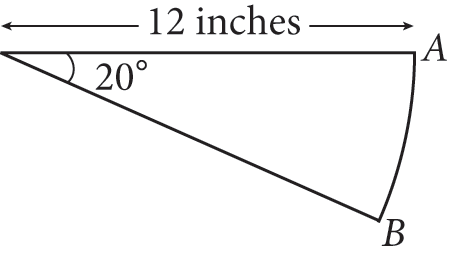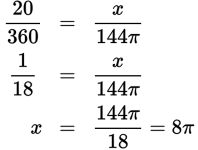# SAT Math Multiple Choice Question 978: Answer and Explanation

### Test Information

Question: 978

14.The figure above shows the path traced by the hand of a scale as it moves from A to B. What is the area, in square inches, of the region passed over by the scale's hand?

• A. 2π
• B. 8π
• C. 12π
• D. 16π

Explanation:

B

Difficulty: Hard

Category: Additional Topics in Math / Geometry

Strategic Advice: Sometimes, the first step to answering a question is thinking logically: If the hand of the scale were to make a full rotation, its path would be a circle. Use this fact and the information provided in the figure to write and solve a proportion to answer the question.

Getting to the Answer: The length of the scale hand is also the radius of a circle created from one full rotation of the hand around the entire scale. Therefore, the full area passed over by the hand of the scale is A = πr2 = π × 122 = 144π. You can use this in conjunction with the degree measures of the sector in the diagram (20°) and the full circle (360°) to find the area of the sector (the area passed over by the scale hand):